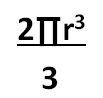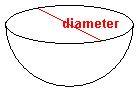Volume of a Hemisphere

Instructor: Nola Bridgens

Nola has taught elementary school and tutored for four years. She has a bachelor's degree in Elementary Education, a master's degree in Marketing, and is a certified teacher.

In this lesson, we will explore the characteristics of a hemisphere and define what volume is. We will also learn the formula to calculate the volume of a hemisphere and try some practice problems.

Party Punch Problem

It is the end of the school year and your class is having a last day of school party. Your teacher has given each student a party task that will draw on what he or she learned in class that year.

You are in charge of the punch. Your teacher gives you a glass punch bowl and tells you to find out how much punch you'll need to fill it exactly to the top. After careful examination, you realize that the punch bowl is half of a sphere, or a hemisphere. You remember from math class that volume is the area inside of an object. Now, all you need to do is recall the formula for volume of a hemisphere to complete your contribution to the party.

Volume of a Hemisphere

A hemisphere is a 3-dimensional object that is half of a sphere. Volume is the amount of space inside of an object. The formula for the volume of a hemisphere is:To apply this formula, you need to know what TT and radius mean:

• TT is the symbol for pi; it is a mathematical constant that is always equal to 3.14.
• The radius is the distance from the center point of a sphere to the circumference, an outside ring. The radius is always half of the diameter.Applying the Formula

Let's look at an example to see how the formula works.

Find the volume of a hemisphere with a radius of 5 ft.

We know that TT=3.14 and r = 5, so we need to plug this information into the formula:

(2)(3.14)(53) / 3 = (6.28)(53) / 3 = (6.28)(125) / 3 = 785 / 3 = 261.67 cubic feet.

The volume of a hemisphere with a radius of 5 feet is 261.67 cubic feet.

To unlock this lesson you must be a Study.com Member.

Register to view this lesson

Are you a student or a teacher?

See for yourself why 30 million people use Study.com

Become a Study.com member and start learning now.
Back
What teachers are saying about Study.com

Earning College Credit

Did you know… We have over 200 college courses that prepare you to earn credit by exam that is accepted by over 1,500 colleges and universities. You can test out of the first two years of college and save thousands off your degree. Anyone can earn credit-by-exam regardless of age or education level.